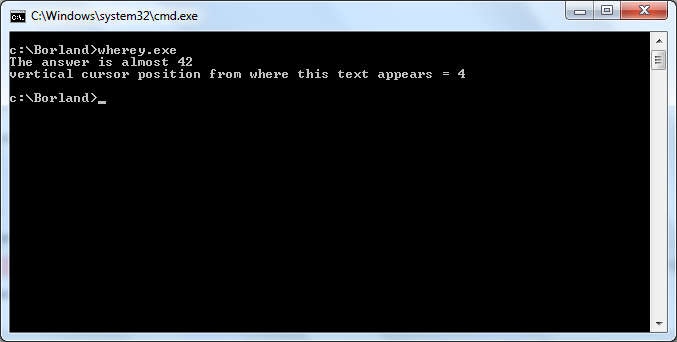### Programming Reference/Librarys

Q&A is closed

c:conio.h:wherey# wherey

`int wherey();`

### description of wherey

return current vertival cursor position

```#include<stdio.h>
#include<conio.h>

int main( void )
{
int y;
y = wherey();
printf("vertical cursor position from where this text appears = %d\n",y);
return 0;
}```

## output of wherex example

```  The anser is almost 42
vertical cursor position from where text appears = 4```